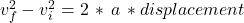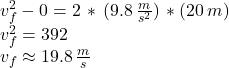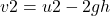## A body is dropped from a height of 30m. What is the velocity of the body after it has covered a distance of 20 m? (Given g= 10 m/s 2) p

Question

A body is dropped from a height of 30m. What is the velocity of the body after it has covered a distance of 20 m? (Given g= 10 m/s 2)

in progress 0
6 months 2021-07-26T12:51:24+00:00 2 Answers 105 views 1

## Answers ( )

approximately 19.8  m/s

If using g = 10 m/s^2, the answer rounds to 20 m/s

Explanation:

You can use the kinematic formula that relates distance covered, velocities and acceleration (without time dependence) given by:which in our case becomes:If using g = 10 m/s^2, the answer is 20 m/s

the velocity is 20m/s

Explanation:

data given

1. Height (h)=20m
2. Gravity (g)=10m/s2
3. velocity v = 0
4. velocity u =?0 = u2 – 2 (10)(20)

0 = u2 – 400

400 = u2

20m/s = u